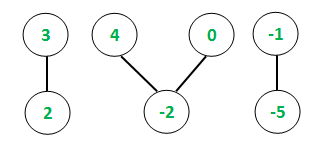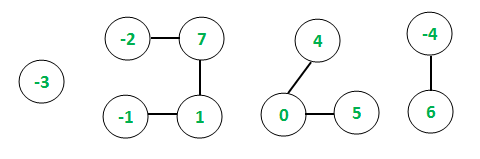# Largest subarray sum of all connected components in undirected graph

Given an undirected graph with V vertices and E edges, the task is to find the maximum contiguous subarray sum among all the connected components of the graph.

Examples:

Input: E = 4, V = 7Output:
Maximum subarray sum among all connected components = 5
Explanation:
Connected Components and maximum subarray sums are as follows:
[3, 2]: Maximum subarray sum = 3 + 2 = 5
[4, -2, 0]: Maximum subarray sum = 4
[-1, -5]: Maximum subarray sum = -1
So, Maximum contiguous subarray sum = 5

Input: E = 6, V = 10Output:
Maximum subarray sum among all connected components = 9
Explanation:
Connected Components and maximum subarray sums are as follows:
[-3]: Maximum subarray sum = -3
[-2, 7, 1, -1]: Maximum subarray sum = 7 + 1 = 8
[4, 0, 5]: Maximum subarray sum = 4 + 0 + 5 = 9
[-4, 6]: Maximum subarray sum = 6
So, Maximum contiguous subarray sum = 9

Approach: The idea is to use Depth First Search Traversal to keep track of the connected components in the undirected graph as explained in this article. For each connected component, the array is analyzed and the maximum contiguous subarray sum is computed based on Kadane’s Algorithm as explained in this article. A global variable is set that is compared at each iteration with the local sum values to obtain the final result.

Below is the implementation of the above approach:

## C++

 `// C++ implementation to find ` `// largest subarray sum among ` `// all connected components ` ` `  `#include ` `using` `namespace` `std; ` ` `  `// Function to traverse the undirected ` `// graph using the Depth first traversal ` `void` `depthFirst(``int` `v, vector<``int``> graph[], ` `                ``vector<``bool``>& visited, ` `                ``vector<``int``>& storeChain) ` `{ ` `    ``// Marking the visited ` `    ``// vertex as true ` `    ``visited[v] = ``true``; ` ` `  `    ``// Store the connected chain ` `    ``storeChain.push_back(v); ` ` `  `    ``for` `(``auto` `i : graph[v]) { ` `        ``if` `(visited[i] == ``false``) { ` ` `  `            ``// Recursive call to ` `            ``// the DFS algorithm ` `            ``depthFirst(i, graph, ` `                       ``visited, storeChain); ` `        ``} ` `    ``} ` `} ` ` `  `// Function to return maximum ` `// subarray sum of each connected ` `// component using Kadane's Algorithm ` `int` `subarraySum(``int` `arr[], ``int` `n) ` `{ ` `    ``int` `maxSubarraySum = arr; ` `    ``int` `currentMax = arr; ` ` `  `    ``// Following loop finds maximum ` `    ``// subarray sum based on Kadane's ` `    ``// algorithm ` `    ``for` `(``int` `i = 1; i < n; i++) { ` `        ``currentMax = max(arr[i], ` `                         ``arr[i] + currentMax); ` ` `  `        ``// Global maximum subarray sum ` `        ``maxSubarraySum = max(maxSubarraySum, ` `                             ``currentMax); ` `    ``} ` ` `  `    ``// Returning the sum ` `    ``return` `maxSubarraySum; ` `} ` ` `  `// Function to find the maximum subarray ` `// sum among all connected components ` `void` `maxSubarraySum( ` `    ``vector<``int``> graph[], ``int` `vertices, ` `    ``vector<``int``> values) ` `{ ` `    ``// Initializing boolean array ` `    ``// to mark visited vertices ` `    ``vector<``bool``> visited(1001, ``false``); ` ` `  `    ``// maxSum stores the ` `    ``// maximum subarray sum ` `    ``int` `maxSum = INT_MIN; ` ` `  `    ``// Following loop invokes DFS algorithm ` `    ``for` `(``int` `i = 1; i <= vertices; i++) { ` `        ``if` `(visited[i] == ``false``) { ` ` `  `            ``// Variable to hold ` `            ``// temporary length ` `            ``int` `sizeChain; ` ` `  `            ``// Variable to hold temporary ` `            ``// maximum subarray sum values ` `            ``int` `tempSum; ` ` `  `            ``// Container to store each chain ` `            ``vector<``int``> storeChain; ` ` `  `            ``// DFS algorithm ` `            ``depthFirst(i, graph, visited, storeChain); ` ` `  `            ``// Variable to hold each chain size ` `            ``sizeChain = storeChain.size(); ` ` `  `            ``// Container to store values ` `            ``// of vertices of individual chains ` `            ``int` `chainValues[sizeChain + 1]; ` ` `  `            ``// Storing the values of each chain ` `            ``for` `(``int` `i = 0; i < sizeChain; i++) { ` `                ``int` `temp = values[storeChain[i] - 1]; ` `                ``chainValues[i] = temp; ` `            ``} ` ` `  `            ``// Function call to find maximum ` `            ``// subarray sum of current connection ` `            ``tempSum = subarraySum(chainValues, ` `                                  ``sizeChain); ` ` `  `            ``// Conditional to store current ` `            ``// maximum subarray sum ` `            ``if` `(tempSum > maxSum) { ` `                ``maxSum = tempSum; ` `            ``} ` `        ``} ` `    ``} ` ` `  `    ``// Printing global maximum subarray sum ` `    ``cout << ``"Maximum subarray sum among all "``; ` `    ``cout << ``"connected components = "``; ` `    ``cout << maxSum; ` `} ` ` `  `// Driver code ` `int` `main() ` `{ ` `    ``// Initializing graph in the ` `    ``// form of adjacency list ` `    ``vector<``int``> graph; ` ` `  `    ``// Defining the number ` `    ``// of edges and vertices ` `    ``int` `E, V; ` `    ``E = 4; ` `    ``V = 7; ` ` `  `    ``// Assigning the values for each ` `    ``// vertex of the undirected graph ` `    ``vector<``int``> values; ` `    ``values.push_back(3); ` `    ``values.push_back(2); ` `    ``values.push_back(4); ` `    ``values.push_back(-2); ` `    ``values.push_back(0); ` `    ``values.push_back(-1); ` `    ``values.push_back(-5); ` ` `  `    ``// Constructing the undirected graph ` `    ``graph.push_back(2); ` `    ``graph.push_back(1); ` `    ``graph.push_back(4); ` `    ``graph.push_back(3); ` `    ``graph.push_back(5); ` `    ``graph.push_back(4); ` `    ``graph.push_back(7); ` `    ``graph.push_back(6); ` ` `  `    ``maxSubarraySum(graph, V, values); ` `    ``return` `0; ` `} `

Output:

```Maximum subarray sum among all connected components = 5
```

Time Complexity: O(V2)
The DFS algorithm takes O(V + E) time to run, where V, E are the vertices and edges of the undirected graph. Further, the maximum contiguous subarray sum is found at each iteration that takes an additional O(V) to compute and return the result based on Kadane’s Algorithm. Hence, the overall complexity is O(V2)My Personal Notes arrow_drop_upCheck out this Author's contributed articles.

If you like GeeksforGeeks and would like to contribute, you can also write an article using contribute.geeksforgeeks.org or mail your article to contribute@geeksforgeeks.org. See your article appearing on the GeeksforGeeks main page and help other Geeks.

Please Improve this article if you find anything incorrect by clicking on the "Improve Article" button below.